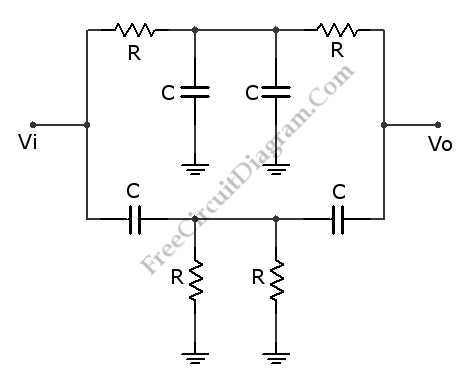## Variable Q Twin-T Notch Filter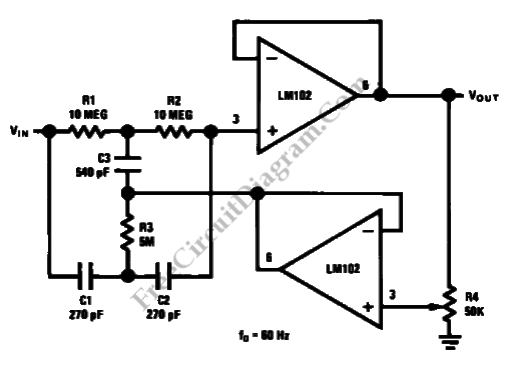This is a Variable Q Twin-T Notch Filter circuit. This circuit is used when low Q is needed. The Lower Q gives some advantages in rejecting the signal where the signal deviate slightly from the null of the notch network. The Q of this circuit can be adjusted from 0.3 to 50. This circuit has two voltage follower. The second […]

## Bootstrapped Twin-T Notch Filter Produces High Q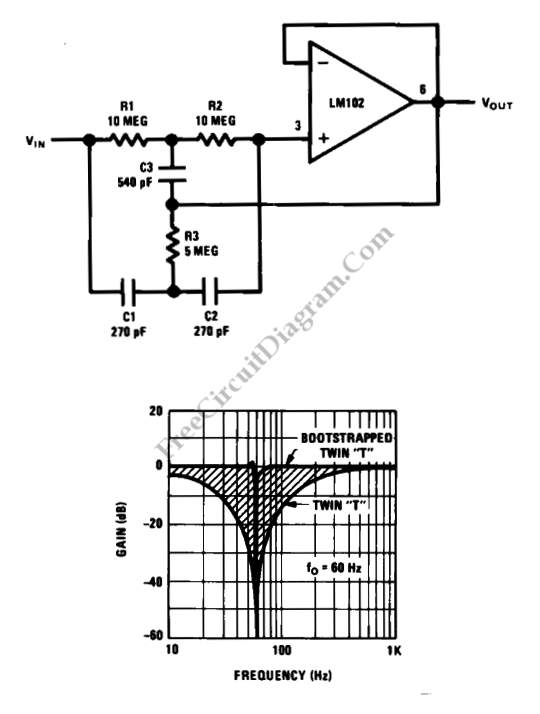This is a Bootstrapped Twin-T Notch Filter circuit. This circuit can generate high Q by combining the twin “T” with LM102 voltage follower. The generated Q is greater than 50. This circuit uses small capacitors since the LM102 uses the large resistance values in the “T”. Beside that, voltage follower also used as buffer that gives high input resistance of […]

## Phase-Shift Oscillator with Inverter Gates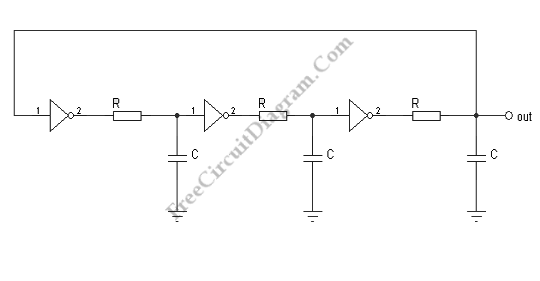The following circuit diagram show us another kind of CMOS oscillator. It’s will always oscillate because its a loop of three inverters. It would oscillate at a very high frequency (MHz) without the RC components depending on the propagation delays of the inverters. The RC branches give a phase shift of 60′ at some frequency, so the overall phase shift […]

## Single Gate Relaxation Oscillator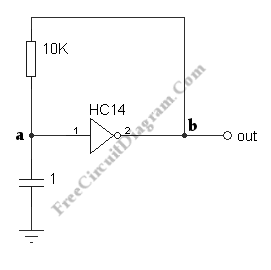Below is a relaxation oscillator circuit using a 74HC14, an inverter with a Schmitt trigger input. It’s mean that the transition point for an increasing input is higher in voltage than the transition point for a decreasing input. The difference is called the “hysteresis.” Such an input is very useful for a slowly-varying inout or one with noise. The opposite […]

## Voltage-Controlled Low-Pass Filter with LM13600 OTA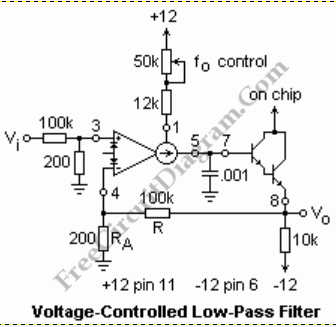Below is a circuit that demonstrates one use of an OTA using an LM13600. Take a note that the strange symbol stand for OTA. In this circuit, negative feedback is employed which is low-pass filter whose corner frequency can be electrically adjusted. We can a filter that would tune from 2700 Hz to 9000 Hz approximately for the values shown. […]

## Varactor Test (Experiment) Circuit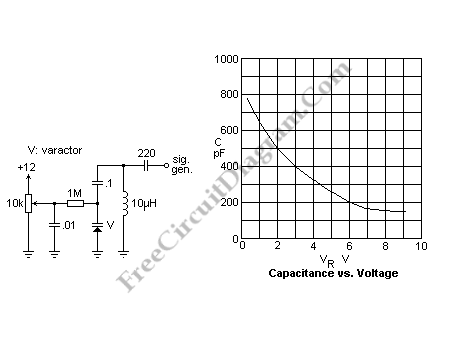A varactor is also known as a varicap or a variable capacitance diode. An electrically controllable capacitance is provided by this varactor which can be used in tuned circuits. What makes it’s advantageous in many application are the small size and inexpensive. If compared to a manually controlled variable capacitor, this varactor has disadvantages such as lower Q, nonlinearity, lower […]